# Rational functions

A rational expression is the ratio of two polynomial expressions. Such expressions arise in many modeling situations. As well, as many facts are known about polynomial expressions, much can be determined about rational expressions. This section covers some additional details that arise when graphing such expressions.

## Rational functions

The rational numbers are simply ratios of integers, of the form $p/q$ for non-zero $q$. A rational function is a ratio of polynomial functions of the form $p(x)/q(x)$, again $q$ is non-zero, but may have zeros.

We know that polynomials have nice behaviors due to the following facts:

• Behaviors at $-\infty$, $\infty$ are known just from the leading term.

• There are possible wiggles up and down, the exact behavior depends on intermediate terms, but there can be no more than $n-1$ wiggles.

• The number of zeros is no more than $n$, the degree of the polynomial.

Rational functions are not quite so nice:

• behavior at $-\infty$ and $\infty$ can be like a polynomial of any degree, including constants

• behaviour at any value x can blow up due to division by 0 - rational functions, unlike polynomials, are not always defined

• The function may or may not cross zero, even if the range includes every other point, as the graph of $f(x) =1/x$ will show.

Here, as with our discussion on polynomials, we are interested for now in just a few properties:

• What happens to $f(x)$ when $x$ gets really big or really small (towards $\infty$ or $-\infty$)?

• What happens near the values where $q(x) = 0$?

• When is $f(x) = 0$?

These questions can often be answered with a graph, but with rational functions we will see that care must be take when producing a useful graph.

For example, consider this graph generated from a simple rational function:

$~ f(x) = \frac{(x-1)^2 \cdot (x-2)}{(x+3) \cdot (x-3)}. ~$

using CalculusWithJulia   # loads the SymPy package
using Plots
f(x) = (x-1)^2 * (x-2) / ((x+3)*(x-3) )
plot(f, -10, 10)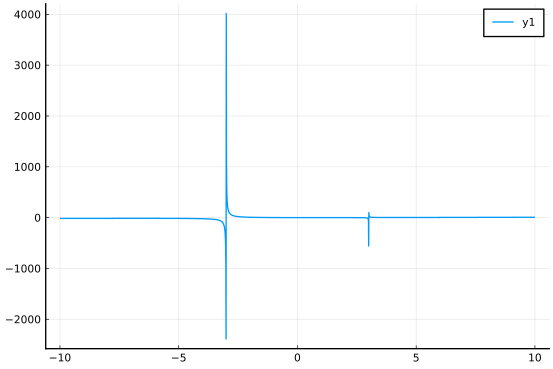We would be hard pressed to answer any of the three questions above from the graph, though, on inspection, we might think the strange spikes have something to do with $x$ values where $q(x)=0$.

The question of big or small $x$ is not answered well with this graph, as the spikes dominate the scale of the $y$-axis. Setting a much larger viewing window illuminates this question:

plot(f, -100, 100)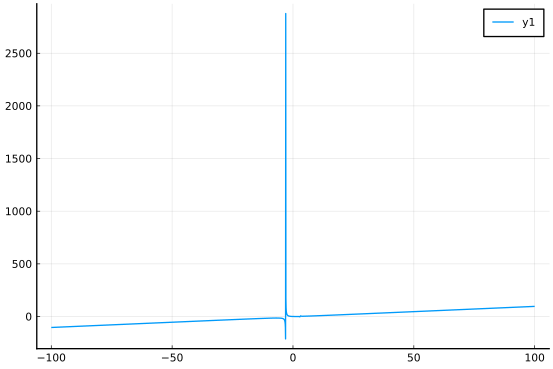We can see from this, that the function eventually looks like a slanted straight line. The eventual shape of the graph is something that can be determined just from the two leading terms.

The spikes haven't vanished completely. It is just that with only a few hundred points to make the graph, there aren't any values near enough to the problem to make a large spike. The spikes happen because the function has a vertical asymptote at these values. Though not quite right, it is reasonable to think of the graph graph being made by selecting a few hundred points in the specified domain, computing the corresponding $y$ values, plotting the pairs, and finally connecting the points with straight line segments. Near a vertical asymptote the function values can be arbitrarily large in absolute values, though at the vertical asymptote the function is undefined. This graph doesn't show such detail.

The spikes will be related to the points where $q(x) = 0$, though not necessarily, as not all such points will produce a vertical asymptote.

Where the function crosses $0$ is very hard to tell from these two graphs. As well, other finer features, such as local peaks or valleys, when present, can be hard to identify as the $y$-scale is set to accommodate the asymptotes. Working around the asymptotes requires some extra effort. Strategies are discussed herein.

## Asymptotes

Formally, an asymptote of a curve is a line such that the distance between the curve and the line approaches $0$ as they tend to infinity. Tending to infinity can happen as $x \rightarrow \pm \infty$ or $y \rightarrow \pm \infty$, the former being related to horizontal asymptotes or slant asymptotes, the latter being related to vertical asymptotes.

### Behaviour as $x \rightarrow \infty$ or $x \rightarrow -\infty$.

Let's look more closely at our example rational function using symbolic math.

In particular, let's rewrite the expression in terms of its numerator and denominator:

@vars x real=true
a =  (x-1)^2 * (x-2)
b = (x+3)*(x-3)

\begin{equation*}\left(x - 3\right) \left(x + 3\right)\end{equation*}

Euclid's division algorithm can be used for polynomials $a(x)$ and $b(x)$ to produce $q(x)$ and $r(x)$ with $a = b\cdot q + r$ and the degree of $r(x)$ is less than the degree of $b(x)$. This is in direct analogy to the division algorithm of integers, only there the value of the remainder, $r(x)$, satisfies $0 \leq r < b$. Given $q(x)$ and $r(x)$ as above, we can reexpress the rational function

$~ \frac{a(x)}{b(x)} = q(x) + \frac{r(x)}{b(x)}. ~$

The rational expression on the right-hand side has larger degree in the denominator.

The division algorithm is implemented in Julia generically through the divrem method:

q, r = divrem(a, b)

(floor((x - 2)*(x - 1)^2/((x - 3)*(x + 3))), -(x - 3)*(x + 3)*floor((x - 2)
*(x - 1)^2/((x - 3)*(x + 3))) + (x - 2)*(x - 1)^2)


This yield the decomposition of a/b:

q + r/b

\begin{equation*}\left\lfloor{\frac{\left(x - 2\right) \left(x - 1\right)^{2}}{\left(x - 3\right) \left(x + 3\right)}}\right\rfloor + \frac{- \left(x - 3\right) \left(x + 3\right) \left\lfloor{\frac{\left(x - 2\right) \left(x - 1\right)^{2}}{\left(x - 3\right) \left(x + 3\right)}}\right\rfloor + \left(x - 2\right) \left(x - 1\right)^{2}}{\left(x - 3\right) \left(x + 3\right)}\end{equation*}

A similar result can be found using the apart function, which can be easier to use if the expression is not given in terms of a separate numerator and denominator.

f(x) = (x-1)^2 * (x-2) / ((x+3)*(x-3))  # as a function
p = f(x)                                # a symbolic expression
apart(p)

\begin{equation*}x - 4 + \frac{40}{3 \left(x + 3\right)} + \frac{2}{3 \left(x - 3\right)}\end{equation*}

This decomposition breaks the rational expression into two pieces: $x-4$ and $40/(3x+9) + 2/(3x-9)$. The first piece would have a graph that is the line with slope $1$ and $y$-intercept $4$. As $x$ goes to $\infty$, the second piece will clearly go towards 0, as this simple graph shows:

plot(apart(p) - (x - 4), 10, 100)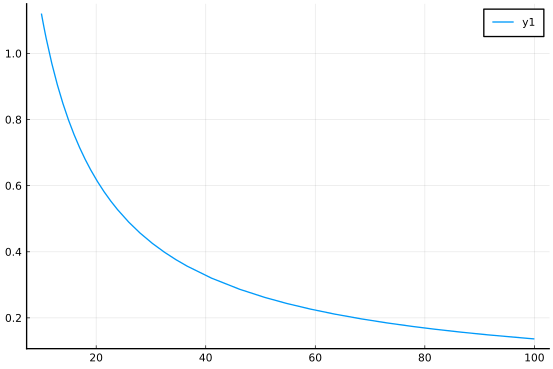Similarly, a plot over $[-100, -10]$ would show decay towards $0$, though in that case from below. Combining these two facts then, it is now no surprise that the graph of the rational function $f(x)$ should approach a straight line, in this case $y=x-4$ as $x \rightarrow \pm \infty$.

We can easily do most of this analysis without needing a computer or algebra. First, we should know that the graph of $y=mx$ is a line with slope $m$, the graph of $y = c$ is a constant line at height $c$, and the graph of $y=c/x^m$, $m > 0$ will decay towards $0$ as $x \rightarrow \pm\infty$. The latter should be clear, as $x^m$ gets big, so its reciprocal goes towards $0$.

The factored form, as $p$ is presented, is a bit hard to work with, rather we use the expanded form, which we get through the cancel function

cancel(p)

\begin{equation*}\frac{x^{3} - 4 x^{2} + 5 x - 2}{x^{2} - 9}\end{equation*}

We can see that numerator is of degree 3 and the denominator of degree $2$. The leading terms are $x^3$ and $x^2$ respectively. If we were to pull those out we would get:

$~ \frac{x^3 \cdot (1 - 4/x + 5/x^2 - 2/x^3)}{x^2 \cdot (1 - 9/x^2)}. ~$

The terms $(1 - 4/x + 5/x^2 - 2/x^3)$ and $(1 - 9/x^2)$ go towards $1$ as $x \rightarrow \pm \infty$, as each term with $x$ goes towards $0$. So the dominant terms comes from the ratio of the leading terms, $x^3$ and $x^2$. This ratio is $x$, so their will be an asymptote of a line with slope $1$. (The fact that the asymptote is $y=x-4$ takes a bit more work, as a division step is needed.)

Just by looking at the ratio of the two leading terms, the behaviour as $x \rightarrow \pm \infty$ can be discerned. If this ratio is of:

• the form $c x^m$ with $m > 1$ then the shape will follow the polynomial growth of of the monomial $c x^m$.

• the form $c x^m$ with $m=1$ then there will be a line with slope $c$ as a slant asymptote.

• the form $cx^0$ with $m=0$ (or just $c$) then there will be a horizontal asymptote $y=c$.

• the form $c/x^{m}$ with $m > 0$ then there will be a horizontal asymptote $y=0$, or the $y$ axis.

To expand on the first points where the degree of the numerator is greater than that of the denominator, we have from the division algorithm that if $a(x)$ is the numerator and $b(x)$ the denominator, then $a(x)/b(x) = q(x) + r(x)/b(x)$ where the degree of $b(x)$ is greater than the degree of $r(x)$, so the rightmost term will have a horizontal asymptote of $0$. This says that the graph will eventually approach the graph of $q(x)$, giving more detail than just saying it follows the shape of the leading term of $q(x)$, at the expense of the work required to find $q(x)$.

##### Examples

Consider the rational expression

$~ \frac{17x^5 - 300x^4 - 1/2}{x^5 - 2x^4 + 3x^3 - 4x^2 + 5}. ~$

The leading term of the numerator is $17x^5$ and the leading term of the denominator is $x^5$. The ratio is $17$ (or $17x^0 = 17x^{5-5}$). As such, we would have a horizontal asymptote $y=17$.

If we consider instead this rational expression:

$~ \frac{x^5 - 2x^4 + 3x^3 - 4x^2 + 5}{5x^4 + 4x^3 + 3x^2 + 2x + 1} ~$

Then we can see that the ratio of the leading terms is $x^5 / (5x^4) = (1/5)x$. We expect a slant asymptote with slope $1/5$, though we would need to divide to see the exact intercept. This is found with

p = (x^5 - 2x^4 + 3x^3 - 4x^2 + 5) / (5x^4 + 4x^3 + 3x^2 + 2x + 1)
apart(p)

\begin{equation*}\frac{x}{5} + \frac{116 x^{3} - 68 x^{2} + 23 x + 139}{25 \left(5 x^{4} + 4 x^{3} + 3 x^{2} + 2 x + 1\right)} - \frac{14}{25}\end{equation*}

The rational function

$~ \frac{5x^3 + 6x^2 + 2}{x-1} ~$

has decomposition $5x^2 + 11x + 11 + 13/(x-1)$:

a = 5x^3 + 6x^2 +2
b = x-1
q, r = divrem(a, b)

(floor((5*x^3 + 6*x^2 + 2)/(x - 1)), 5*x^3 + 6*x^2 - (x - 1)*floor((5*x^3 +
6*x^2 + 2)/(x - 1)) + 2)


The graph of $a/b$ has nothing in common with the graph of $g$ for small $x$

plot(a/b, -3, 3)
plot!(q, -3, 3)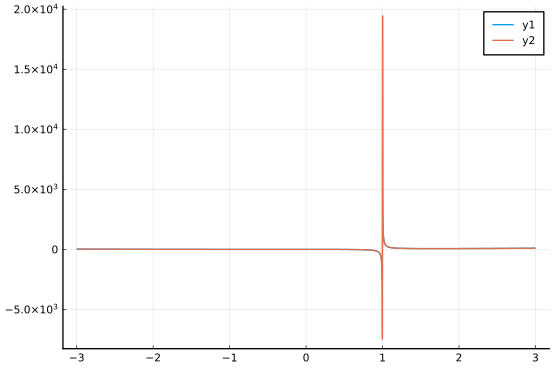But the graphs do match for large $x$:

plot(a/b, 5, 10)
plot!(q, 5, 10)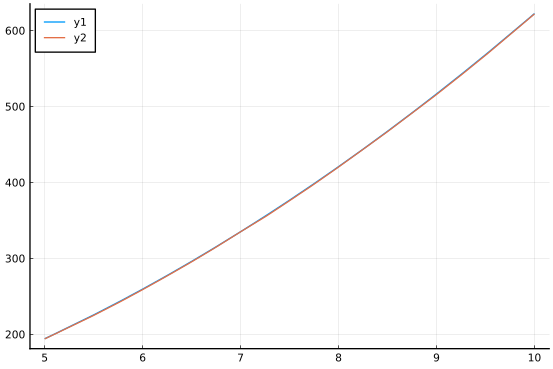Finally, consider this rational expression in factored form:

$~ \frac{(x-2)^3\cdot(x-4)\cdot(x-3)}{(x-5)^4 \cdot (x-6)^2}. ~$

By looking at the powers we can see that the leading term of the numerator will the $x^5$ and the leading term of the denominator $x^6$. The ratio is $1/x^1$. As such, we expect the $y$-axis as a horizontal asymptote:

#### Partial fractions

The apart function was useful to express a rational function in terms of a polynomial plus additional rational functions whose horizontal asymptotes are $0$. This function computes the partial fraction decomposition of a rational function. Outside of the initial polynomial, this decomposition is a reexpression of a rational function into a sum of rational functions, where the denominators are irreducible, or unable to be further factored (non-trivially) and the numerators have lower degree than the denominator. Hence the horizontal asymptotes of $0$.

To see another example we have:

x = symbols("x")
p = (x-1)*(x-2)
q = (x-3)^3 * (x^2 - x - 1)
apart(p/q)

\begin{equation*}\frac{2 x - 1}{25 \left(x^{2} - x - 1\right)} - \frac{2}{25 \left(x - 3\right)} + \frac{1}{5 \left(x - 3\right)^{2}} + \frac{2}{5 \left(x - 3\right)^{3}}\end{equation*}

The denominator, $q$, has factors $x-3$ and $x^2 - x - 1$, each irreducible. The answer is expressed in terms of a sum of rational functions each with a denominator coming from one of these factors, possibly with a power.

### Vertical asymptotes

As just discussed, the graph of $1/x$ will have a horizontal asymptote. However it will also show a spike at $0$:

plot(1/x, -1, 1)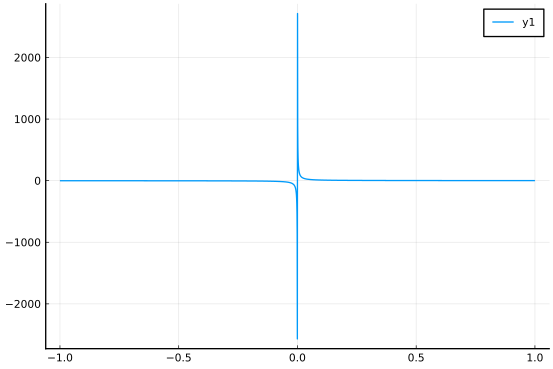Again, this spike is an artifact of the plotting algorithm. The $y$ values for $x$-values just smaller than $0$ are large negative values and the $x$ values just larger than $0$ produce large, positive $y$ values. The two points with $x$ components closest to $0$ are connected with a line, though that is misleading.

The line $x = 0$ is a vertical asymptote for the graph of $1/x$. As $x$ values get close to $0$ from the right, the $y$ values go towards $\infty$ and as the $x$ values get close to $0$ on the left, the $y$ values go towards $-\infty$.

This has everything to do with the fact that $0$ is a root of the denominator.

For a rational function $p(x)/q(x)$, the roots of $q(x)$ may or may not be asymptotes. For a root $c$ if $p(c)$ is not zero then the line $x=c$ will be a vertical asymptote. If $c$ is a root of both $p(x)$ and $q(x)$, then we can rewrite the expression as:

$~ \frac{p(x)}{q(x)} = \frac{(x-c)^m r(x)}{(x-c)^n s(x)}, ~$

where both $r(c)$ and $s(c)$ are non zero. Knowing $m$ and $n$ (the multiplicities of the root $c$) allows the following to be said:

• If $m < n$ then $x=c$ will be a vertical asymptote.

• If $m \geq n$ then $x=c$ will not be vertical asymptote. (The value $c$ will be known as a removable singularity). In this case, the graph of $p(x)/q(x)$ and the graph of $(x-c)^{m-n}r(x)/s(x)$ will differ, as the latter will include a value for $x=c$, whereas $x=c$ is not in the domain of $p(x)/q(x)$.

Finding the multiplicity may or may not be hard, but there is a quick check that is often correct. With Julia, if you have a rational function that has f(c) evaluate to Inf or -Inf then there will be a vertical asymptote. If the expression evaluates to NaN, more analysis is needed. (The value of 0/0 is NaN, where as 1/0 is Inf.)

For example, the function $f(x) = ((x-1)^2 \cdot (x-2)) / ((x+3) \cdot (x-3))$ has vertical asymptotes at $-3$ and $3$, as its graph illustrated. Without the graph we could see this as well:

f(x) = (x-1)^2 * (x-2) / ((x+3)*(x-3) )
f(3), f(-3)

(Inf, -Inf)


#### Graphing with vertical asymptotes

As seen in several graphs, the basic plotting algorithm does a poor job with vertical asymptotes. For example, it may erroneously connect their values with a steep vertical line, or the $y$-axis scale can get so large as to make reading the rest of the graph impossible. There are some trick to work around this.

Consider again the function $f(x) = ((x-1)^2 \cdot (x-2)) / ((x+3) \cdot(x-3))$. Without much work, we can see that $x=3$ and $x=-3$ will be vertical asymptotes and there will be a slant asymptote with slope 1. How to graph this?

We can avoid the vertical asymptotes in our viewing window. For example we could look at the area between the vertical asymptotes, by plotting over $(-2.9, 2.9)$, say:

f(x) = (x-1)^2 * (x-2) / ((x+3)*(x-3) )
plot(f, -2.9, 2.9)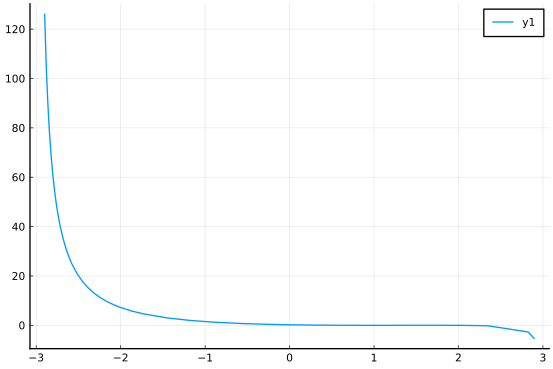This backs off by $\delta = 0.1$. As we have that $2.9 - 3$ is $\delta$ and $1/\delta$ is 10, the $y$ axis won't get too large, and indeed it doesn't.

This graph doesn't show well the two zeros at $x=1$ and $x=2$, for that a narrower viewing window is needed. By successively panning throughout the interesting part of the graph, we can get a view of the function.

We can also clip the y axis. The plot function can be passed an argument ylims=(lo, hi) to limit which values are plotted. With this, we can have:

plot(f, -5, 5, ylims=(-20, 20))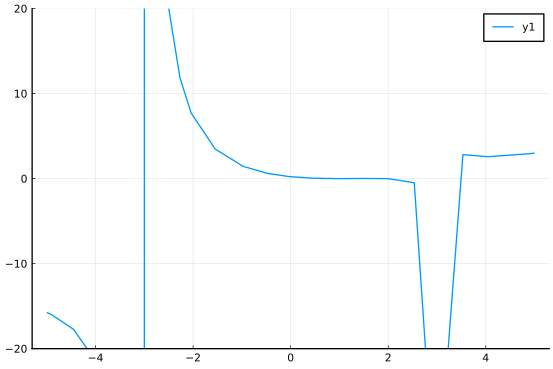This isn't ideal, as the large values are still computed, just the viewing window is clipped. This leaves the vertical asymptotes still effecting the graph.

There is another way, we could ask Julia to not plot $y$ values that get too large. This is not a big request. If instead of the value of f(x) - when it is large - -we use NaN instead, then the connect-the-dots algorithm will skip those values.

This is actually quite easy to implement, if we operate on the function object. Here is a possible trimplot function:

function trimplot(f, a, b, c=20; kwargs...)
fn = x -> abs(f(x)) < c ? f(x) : NaN
plot(fn, a, b; kwargs...)
end


As before, the x -> of the function indicates that this will return an anonymous function, which does what we want: replace large values with NaN.

A more robust version of such a function is in the CalculusWithJulia package, so the above definition need not be used.

Now to graph:

trimplot(f, -25, 25, 30)


We can see the general shape of 3 curves broken up by the vertical asymptotes. The two on the side heading off towards the line $x-4$ and the one in the middle. We still can't see the precise location of the zeros, but that wouldn't be the case with most graphs that show asymptotic behaviors. However, we can clearly tell where to "zoom in" were those of interest.

### Sign charts

When sketching graphs of rational functions by hand, it is useful to use sign charts. A sign chart of a function indicates when the function is positive, negative, $0$, or undefined. It typically is represented along the lines of this one for $f(x) = x^3 - x$:

    -    0   +   0   -   0   +
< ----- -1 ----- 0 ----- 1 ----- >

The usual recipe for construction follows these steps:

• Identify when the function is $0$ or undefined. Place those values on a number line.

• Identify "test points" within each implied interval (these are $(-\infty, -1)$, $(-1,0)$, $(0,1)$, and $(1, \infty)$ in the example) and check for the sign of $f(x)$ at these test points. Write in -, +, 0, or *, as appropriate. The value comes from the fact that "continuous" functions may only change sign when they cross $0$ or are undefined.

With the computer, where it is convenient to draw a graph, it might be better to emphasize the sign on the graph of the function. This helper function will do so, plotting the function and then coloring it different colors based on the sign of $f(x)$:

function signchart(f, a, b)
xs = range(a, stop=b, length=200)
ys = f.(xs)
cols = [fx < 0 ? :red  : :blue for fx in ys]
plot(xs, ys, color=cols, linewidth=5, legend=false)
plot!(zero, a, b)
end


(The function is defined in CalculusWithJulia, so need not be typed in.)

f(x) = x^3 - x
signchart(f, -3/2, 3/2)


## Pade approximate

One area where rational functions are employed is in approximating functions. Later, the Taylor polynomial will be seen to be a polynomial that approximates well a function (where "well" will be described later). The Pade approximation is similar, though uses a rational function for the form $p(x)/q(x)$, where $q(0)=1$ is customary.

Some example approximations are

$~ \sin(x) \approx \frac{x - 7/60 \cdot x^3}{1 + 1/20 \cdot x^2} ~$

and

$~ \tan(x) \approx \frac{x - 1/15 \cdot x^3}{1 - 2/5 \cdot x^2} ~$

We can look graphically at these approximations:

sin_p(x) = (x - (7/60)*x^3) / (1 + (1/20)*x^2)
tan_p(x) = (x - (1/15)*x^3) / (1 - (2/5)*x^2)
plot(sin, -pi, pi)
plot!(sin_p, -pi, pi)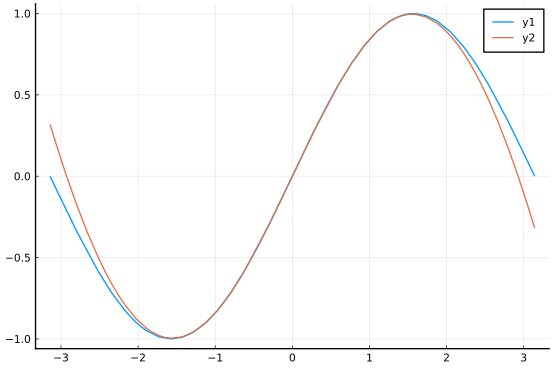plot(tan, -pi/2 + 0.2, pi/2 - 0.2)
plot!(tan_p, -pi/2 + 0.2, pi/2 - 0.2)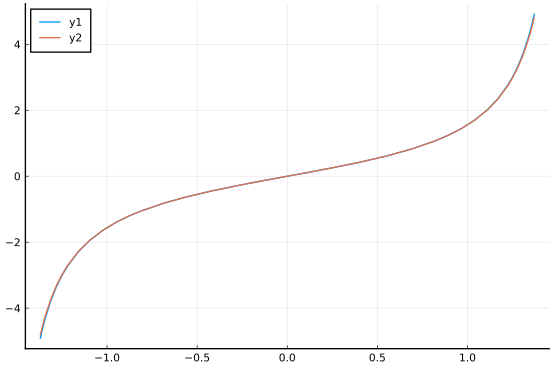## Questions

###### Question

The rational expression $(x^3 - 2x + 3) / (x^2 - x + 1)$ would have

###### Question

The rational expression $(x^2 - x + 1)/ (x^3 - 2x + 3)$ would have

###### Question

The rational expression $(x^2 - x + 1)/ (x^2 - 3x + 3)$ would have

###### Question

The rational expression

$~ \frac{(x-1)\cdot(x-2)\cdot(x-3)}{(x-4)\cdot(x-5)\cdot(x-6)} ~$

would have

###### Question

The rational expression

$~ \frac{(x-1)\cdot(x-2)\cdot(x-3)}{(x-4)\cdot(x-5)\cdot(x-6)} ~$

would have

###### Question

The rational expression

$~ \frac{x^3 - 3x^2 + 2x}{3x^2 - 6x + 2} ~$

has a slant asymptote. What is the equation of that line?

###### Question

Look at the graph of the function $f(x) = ((x-1)\cdot(x-2)) / ((x-3)\cdot(x-4))$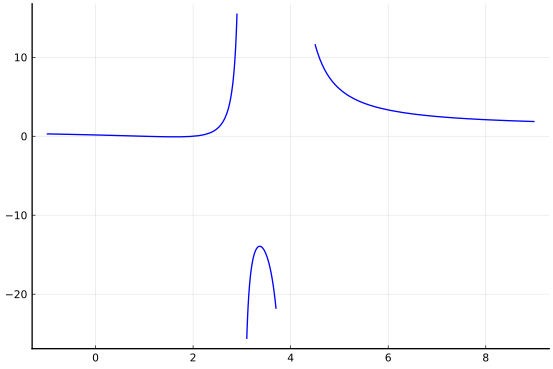Is the following common conception true: "The graph of a function never crosses its asymptotes."

(The wikipedia page indicates that the term "asymptote" was introduced by Apollonius of Perga in his work on conic sections, but in contrast to its modern meaning, he used it to mean any line that does not intersect the given curve. It can sometimes take a while to change perception.)

###### Question

Consider the two graphs of $f(x) = 1/x$ over $[10,20]$ and $[100, 200]$: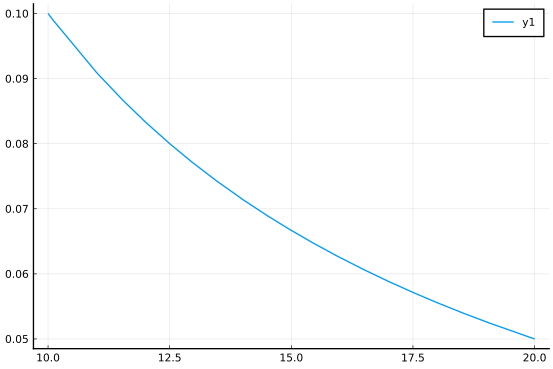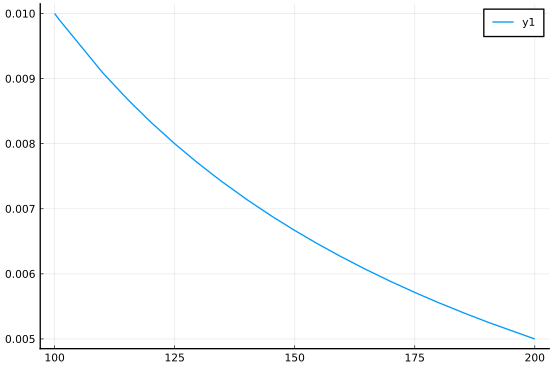The two shapes are basically identical and do not look like straight lines. How does this reconcile with the fact that $f(x)=1/x$ has a horizontal asymptote $y=0$?

###### Question

The amount of drug in a bloodstream after $t$ hours is modeled by the rational function

$~ r(t) = \frac{50t^2}{t^3 + 20}, \quad t \geq 0. ~$

What is the amount of the drug after $1$ hour?

What is the amount of drug in the bloodstream after 24 hours?

What is more accurate: the peak amount is

This graph has

###### Question

The (low-order) Pade approximation for $\sin(x)$ was seen to be $(x - 7/60 \cdot x^3)/(1 + 1/20 \cdot x^2)$. The graph showed that this approximation was fairly close over $[-\pi, \pi]$. Without graphing would you expect the behaviour of the function and its approximation to be similar for large values of $x$?

Why?